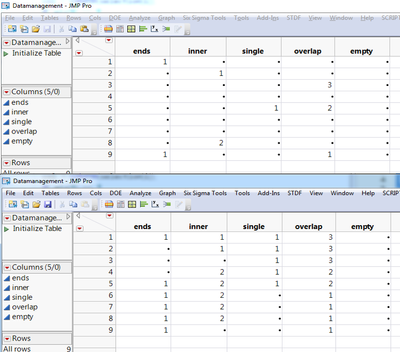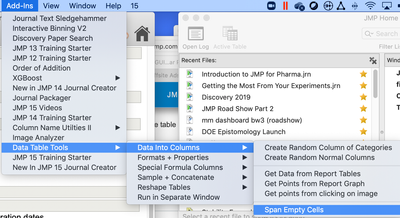It’s World Statistics Day! To honor the theme of the day, the JMP User Community is having conversations about the importance of trust in statistics and data. And we want to hear from you! Tell us the steps you take to ensure that your data is trustworthy.
Choose Language Hide Translation Bar

## Fill in Missing Cells

Report tables often end up having incomplete columns where labels need to be copied to the sequential cells below until the next label where the process is repeated again and again. The Excel Import Wizard has an option to deal with spans of missing cells that are merged. This script can be used after the data is imported and it doesn't require the spans of missing cells to be merged.

I've been using this script, or versions of it, for so long that I'm not sure where the bones of it came from.

I wonder if one could do this without Expressions. For one single column "MyCol1" you could do sth. like this:

For Each Row( If( Is Missing( MyCol1 ), :MyCol1= Lag( MyCol1, 1 ) ) );

Using an automated approach to select the columns like you do combined with this should be able to do.

Making the script into an add-in or putting it into a menu option is my crutch for forgetting the formulas to make it happen,

This is another easy formula to do this.

:Complete is the new column where the formula lives.

:Missing is the source column with missing rows.

//Rows with Character

If( :Missing == "",

:Complete[Row() - 1, Empty()],

:Missing)

//Rows with Numbers

If( Is Missing( :Missing ) == 1,

:Complete [Row() - 1, Empty()],

:Missing)

I'm updateing this add in with this script. Thanks to Brady Brady for some tweaks that make this run faster on really big tables. On a 5MM row table with something to fill in every 10K rows it takes 10-15 sec.

``````Names Default To Here( 1 );
dt = Current Data Table();

dtcollist = dt << get column names;

dlg = Dialog( "Select Column", vlist( selection = List Box( dtcollist ), button( "OK" ) ) );
Remove From( dlg, 2 );
Eval List( dlg );

dtMat = (dt << Get All Columns As Matrix);

For( i = 1, i <= N Items( selection ), i++,
colNum = Contains( dtColList, Name Expr( selection[i] ) );
vMat = dtMat[0, colNum];
mis = Loc( Is Missing( vMat ) );
If( N Row( mis ),
col = column(selection[i]);
If( mis == 1,
col = col[(Loc( vMat ))]
);

For( j = if(mis==1, 2, 1), j <= N Row( mis ), j++,
col[mis[j]] = col[mis[j] - 1]
);
);
);
``````

A similar issue, but a bit different is the case where you want a certain number of cells before the actual entry to have the same value. There you need to make adjustments to not overwrite the previous e.g. 4 cells in case there is a nonmissing entry. Attached a script and a screenshot of the datatable before and after the script has been run. In this script I only covered the numeric case.

``````Names Default To Here( 1 );
// Get Current Data Table
dt = Current Data Table();

// Get Column Names from selection box (where you want to fill)
dtcollist = dt << get column names;
dlg = Dialog(
"Select Column",
vlist( selection = List Box( dtcollist ), button( "OK" ) )
);
Remove From( dlg, 2 );
Eval List( dlg );

// Get matrix of values in selected columns
dtMat = (dt << Get All Columns As Matrix);

// For loop to loop through all selected columns
For( i = 1, i <= N Items( selection ), i++,
// get i-th selected column
colNum = Contains( dtColList, Name Expr( selection[i] ) );
// get values as matrix
vMat = dtMat[0, colNum];
// get rows where matrix is non-empty
mis = Loc( vMat );
// If there is non-missing value loop through previous rows
If( N Row( mis ),
// Get i-th Column name
col = Column( selection[i] );
/*
If( mis == 1,
col = col[(Loc( vMat ))]
);
*/
// Loop to fill previous 4 rows from the row with the k-th non-missing value
For( k = 1, k <= N Rows(mis), k++,
For( j = If(mis[k]-4 < 1, 1,mis[k]-4), j < mis[k], j++,
If(Is Missing(col[j]), col[j] = col[mis[k]],col[j])
);
);
);
);

``````Data Table before and after applying the script

Another update:

This add-in has a better version of the script above plus a whole lot more.

Cheers,

Byron

I have a data table with 2 columns. Need to check if Value is blank for def,jkl,mno and stu and fill in "NA" for these entries.

Any help is appreciated.

 Parameter Value abc 5 def ghi 22 jkl mno pqr 3 stu vwx 73 yz 99

Do you want a script to do this? Interactive you could use recode and save a formula column, this formula script can be used to do what you want

another way is to use a new formula column and in the formula editor you can use the conditional if statement and check for missing ness and set the value to na

then you could use the ctrl+f to search and replace.

Sonthere are many options you can choose from. I like the recode quite much. In scripting I’d probably use another way: save the column in a matrix or list as you want to use nonnumeric values “na” which will change the values to characters, change the missing values to na and then write Back the values to the column values. Hope one of these ideas will help you further

I am trying to write a script for this.The issue here is I might have many more blank columns.But just need to check values for these 4 columns and fill in with "NA".

Thanks

Still stuck at this point not knowing how to proceed further.

Any inputs appreciated!

Thanks

Hi,

thow there might be a more elegant version these two options should work.

Attached is a data table including a tavble script doing what you want (I think). In addition there is a Column with a formula using the Match statement doing the same. The script I used is

``````Names Default to Here(1);
dt = Current Data Table();
For(i = 1, i <= N Rows(dt), i++,
n = dt << get rows where(:Parameter == "def");
Column("Value")[n] = "NA";
n = dt << get rows where(:Parameter == "jkl");
Column("Value")[n] = "NA";
n = dt << get rows where(:Parameter == "mno");
Column("Value")[n] = "NA";
n = dt << get rows where(:Parameter == "d");
Column("Value")[n] = "NA";
);``````

Best,

Martin

I have a case where I want to fill values from multiple columns forwards and backwards within chunks of the table based on a label column. After all this discussion, I don't think there is an elegant way to do that -- please correct if I'm wrong.

I must say, these are some horribly convoluted solutions for what should be a 1-liner:

https://pandas.pydata.org/pandas-docs/stable/reference/api/pandas.core.groupby.DataFrameGroupBy.fill...nearly sure that the span empty cells tool will do what you're looking for.

@bayesfactor wrote:
I must say, these are some horribly convoluted solutions for what should be a 1-liner:

I agree there is just one line of code the user must write in the link you provided, however the underlying function is not a oneliner. This is true for most of the easy to use functions in almost any programming language, if it is R, Python, MATLAB, even in C/C++, ....

In the data table tools add-in (as @Byron_JMP mentioned) you will find a functionality Span Empty Cells, where @brady_brady has created such a function you could use to do what you want with one command. Though the tool put the selection of the table and the columns and all settings into an interface. So if you would like to do it differently you would have to modify the function source code so it takes the selected columns and accepts a seperate inpout specifying the way to span the cells.

In case you want to have a JSL function to do this to be able to incorporate it in other scripts, it would be a good idea to put this request both to support@jmp.com and the JMP Wish List.

The other way is to build on Brady's scripted function and modifying the routine to be used as a JSL function in a JSL script.

Article Labels

There are no labels assigned to this post.

Article Tags